## A metal block of mass 3 kg is falling downward and has velocity of 0.44 m/s when it is 0.8 m above the floor. It strikes the top of a relaxe

Question

A metal block of mass 3 kg is falling downward and has velocity of 0.44 m/s when it is 0.8 m above the floor. It strikes the top of a relaxed vertical spring of length 0.4 m. The spring constant is 2000 N/m. After striking the spring, the block rebounds. What is the maximum height above the floor that the block reaches after the impact

in progress 0
6 months 2021-07-20T21:42:46+00:00 2 Answers 5 views 0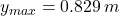Explanation:

Let assume that one end of the spring is attached to the ground. The speed of the metal block when hits the relaxed vertical spring is: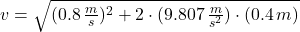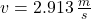The maximum compression of the spring is calculated by using the Principle of Energy Conservation: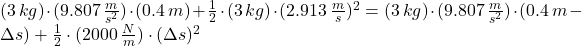After some algebraic handling, a second-order polynomial is formed: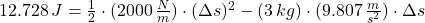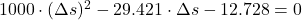The roots of the polynomial are, respectively: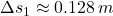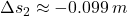The first root is the only solution that is physically reasonable. Then, the elongation of the spring is: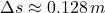The maximum height that the block reaches after rebound is: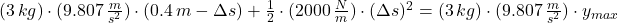0.81 m

Explanation:

In all moment, the total energy is constant:

Energy of sistem = kinetics energy + potencial energy = CONSTANT

So, it doesn’t matter what happens when the block hit the spring, what matters are the (1) and (2) states:

(1): metal block to 0.8 m above the floor

(2): metal block above the floor, with zero velocity ( how high, is the X)

Then: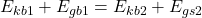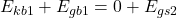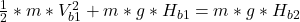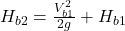Replacing data: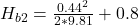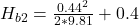HB2 ≈ 0.81 m